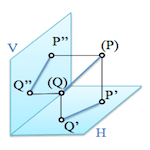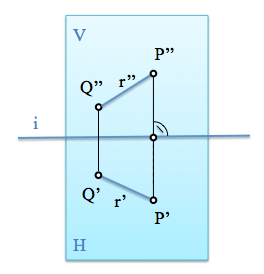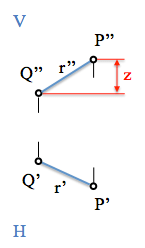# Diédrico System: Projection of the lineAfter seeing the System fundamentals Diédrico, with the projection of a point on two planes orthogonal projection, let's see how you can wean the system of land line as we have two or more points.

This system called “Free System” is more flexible than the traditional because Monge, giving prominence to the reference lines and guiding the model towards a more conceptual spatial geometry; the model is based on understanding the application of the Pythagorean relations and elementary projective, avoiding relationships constructivist.

Gaspard Monge (9 May 17461 – 28 July 1818) was a French mathematician, inventor of descriptive geometry. (W)The projection of a line is cut in two of its points. The figure obtained projections P and Q such points as we have seen above.

Then, following the model set, has befallen the horizontal plane about the vertical plane to determine the dihedral projection of these points and consequently of the line that contains.

Although initially used for concrete flat projections, see what we can wean the result of the position of these so will the concept make sense landline.

We shall be interested only for the representation of the direction projection planes, and not its actual position in space.Consider the projection of the line in the first conventional dihedral system and purge the land line later.

Again we look to bring down planes, pivot axis with the horizontal plane intersection with vertical, straight i, that the projected points on both projections relacinan together by a spectivity.

Projections at each point are reference lines that are perpendicular to this straight intersection (ground line). Namely, line Q’-Q” and P’-P” are perpendicular to i.If we project on parallel planes, for example in two horizontal planes, projections are identical.

The absolute distance of the points to their projections, for example (P)-P’ depends on the concrete level is used for projection but the difference in distances obtained between two points on a line (relative distance) will remain unchanged.

Figure this distance is the tagged value as “z” and is the difference between the distance (P)-P’ and (Q)-Q’, absolute distances of the ends of the straight (segment) to the projection plane (regardless of which of the two planes of the figure is the one used for the screening).

By rotating the horizontal plane the dihedral projections we again. In the picture is shown the difference in height ( valor with)If we eliminate the land line we lose information about the object's shape, obtaining a simplified representation.Indeed, The information provided allows us to restore the line in space.

For the true extent of the segment only need to construct a right triangle in which the hypotenuse is the desired magnitude. The two legs are required, as seen in Figure, height difference, z, and the projection on the plane, r’.

The three relative coordinates are obtained in the direction of the coordinate axes of the trihedral. In the projection will be:With these relative coordinates we see that we can restore the coordinates of the straight line without ground. We will see below some illustrative examples.